# Algebra and Geometry in Electronics

## Magnetic field near a bar magnet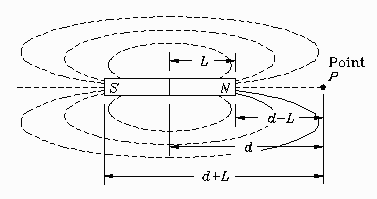Consider the bar magnet shown. The magnetic field strength H at a test point P along the axis of the magnet is given by the formula: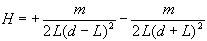This formula was deduced from the following considerations. Imagine a north pole (a monopole) located at test point P. The north pole of the bar magnet repels the test monopole and the south pole attracts it. The first term of the formula, with a + sign, represents the field of the repulsive north pole, and the second term, with a - sign, the attractive south pole. The magnetic field due to either pole falls off as the inverse square of the distance of the test point from the pole.

### Problem:

Show that at large distances H falls off as the inverse cube of the distance.

### Solution

The phrase "at large distances" means that the distance d becomes very large; much larger than the distance L. If we let d become very large in the original expression:we simply get:

H = + 0 - 0

sinced is in the denominator. This is of no help. What is required is to combine the two fractions into one. Start by putting them over a common denominator, like this: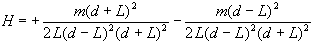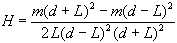Now expand the numerator: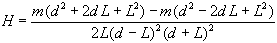and simplify: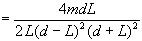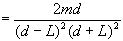We can simplify further by noticing that the denominator can be written as a difference of squares, squared: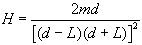or finally: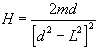In this final expression we can see that if d is much larger than L then we can neglect the L2 inside the square brackets and get the following: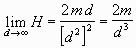This proves that at large distances H falls off as the inverse cube of the distance.

## Unknown resistors in series and parallel

### Problem:

Two resistors, call them R1 and R2, have a resistance of 10 ohms when placed in series and 1.82 ohms when placed in parallel. What are the individual values of R1 and R2?

### Solution

The formula for resistors in series gives:

R1 + R2 = 10.

The formula for resistors in parallel gives: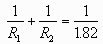or making a single fraction: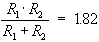These equations form a simultaneous system of equations because the solutions R1 and R2 must satisfy both equations simultaneously. One method to solve this system is to solve the first equation for say R1:

R1 = 10 - R2

and to then substitute this expression for R1 into the second equation wherever R1 occurs: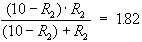This is called the method of substitution. We can now solve this equation for R2. Clearing the denominator and getting everything to the left-hand-side gives:

(10 - R2) · R2 - 18.2 = 0

or

R22 - 10 R2 + 18.2 = 0

Solving this by the quadratic formula, we get: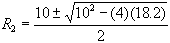The two solutions are R2 = 7.61 and R2 = 2.39. To get a value for R1 we can go back to the series equation:

R1 + R2 = 10.

This equation tells us that if we let R2=7.61 ohms then R1=2.39 ohms. And if we let R2=2.39 ohms then R1=7.61 ohms.Written by Eric Hiob, September 27, 1997 - 3:02:13 PM